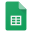# Lattices

## Fall 2019

When: Sundays 13-16, Where: Check Point 420.

Instructors: Barak Weiss, Muli Safra, Nir Bitansky.

About the course: Lattices are intriguing and powerful mathematical objects that have found applications in various areas of computer science, most notably in the areas of cryptography and combinatorial optimization. This course will touch various topics in the theory of lattices, including mathematical basics and advanced aspects, algorithms, complexity, and cryptographic applications.

Prerequisites: The course is meant for graduate CS and Math students (undergrads may join with approval from one of the instructors). There are no formal prerequisites. However, basic concepts from topology, measure theory, and complexity, will be used and if needed informally covered on the fly (with further references given upon request). Perhaps the most important prerequisite is mathematical maturity.

Materials:

• J. H. Conway and N. Sloane, Sphere packings, Lattices and Groups. This is the bible for world records on coverings, packings, and connections to coding theory. Includes very detailed information on the magical Leech lattice.
• Gruber and C. G. Lekkerkerker, Geometry of Numbers. Everything you always wanted to know about lattices but were afraid to ask.
• J. W. S. Cassels, An introduction to the Geometry of Numbers, a gentle introduction with many of the main results.
• C. L. Siegel, Lectures on the Geometry of Numbers, Lectures by the master, includes a treatment of the natural measure on the space of lattices.

Exercises:Untitled spreadsheet

Topics: Below is a tentative list of topics that will be covered.

The mathematical basics behind lattices:

- covolume of a lattice

- Minkowski: first and second theorems

- primitive vectors and primitive sublattices

- Minkowski and Korkine-Zolotarev reduction of a lattice.

- Harder-Narasimhan filtration

- Space of lattices, Mahler compactness criterion, linear action, Haar measure

- Hecke correspondence

- Siegel summation formula

- Application to shortest vector problem

- Rogers formula, applications to shortest vector problem

Computational Complexity

- Classical computational problems on lattices

- The LLL algorithm

- Worst-case to average-case reductions

Cryptographic Applications

- Hashing and Encryption

- The Learning with Errors Problem

- Computing over Encrypted Data